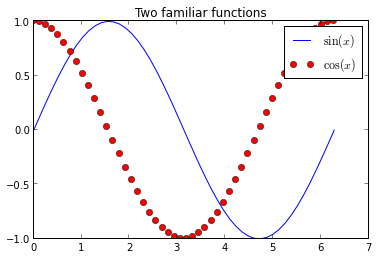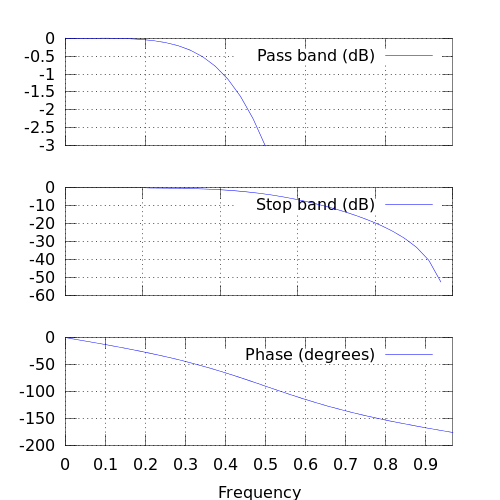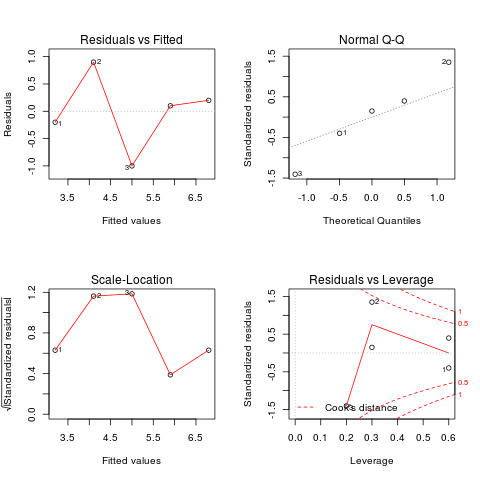Update: made full github repo for blog-as-notebooks, and updated instructions on how to more easily configure everything and use the newest nbconvert for a more streamlined workflow.

Since the notebook was introduced with IPython 0.12, it has proved to be very popular, and we are seeing great adoption of the tool and the underlying file format in research and education. One persistent question we've had since the beginning (even prior to its official release) was whether it would be possible to easily write blog posts using the notebook. The combination of easy editing in markdown with the notebook's ability to contain code, figures and results, makes it an ideal platform for quick authoring of technical documents, so being able to post to a blog is a natural request.

Today, in answering a query about this from a colleague, I decided to try again the status of our conversion pipeline, and I'm happy to report that with a bit of elbow-grease, at least on Blogger things work pretty well!

This post was entirely written as a notebook, and in fact I have now created a github repo, which means that you can see it directly rendered in IPyhton's nbviewer app.

The purpose of this post is to quickly provide a set of instructions on how I got it to work, and to test things out. Please note: this requires code that isn't quite ready for prime-time and is still under heavy development, so expect some assembly.

## Converting your notebook to html with nbconvert¶

The first thing you will need is our nbconvert tool that converts notebooks across formats. The README file in the repo contains the requirements for nbconvert (basically python-markdown, pandoc, docutils from SVN and pygments).

Once you have nbconvert installed, you can convert your notebook to Blogger-friendly html with:

nbconvert -f blogger-html your_notebook.ipynb



This will leave two files in your computer, one named your_notebook.html and one named your_noteboook_header.html; it might also create a directory called your_notebook_files if needed for ancillary files. The first file will contain the body of your post and can be pasted wholesale into the Blogger editing area. The second file contains the CSS and Javascript material needed for the notebook to display correctly, you should only need to use this once to configure your blogger setup (see below):

# Only one notebook so far
(master)longs[blog]> ls
120907-Blogging with the IPython Notebook.ipynb  fig/  old/

# Now run the conversion:
(master)longs[blog]> nbconvert.py -f blogger-html 120907-Blogging\ with\ the\ IPython\ Notebook.ipynb

# This creates the header and html body files
(master)longs[blog]> ls
120907-Blogging with the IPython Notebook_header.html  fig/
120907-Blogging with the IPython Notebook.html         old/
120907-Blogging with the IPython Notebook.ipynb

## Configuring your Blogger blog to accept notebooks¶

The notebook uses a lot of custom CSS for formatting input and output, as well as Javascript from MathJax to display mathematical notation. You will need all this CSS and the Javascript calls in your blog's configuration for your notebook-based posts to display correctly:

1. Once authenticated, go to your blog's overview page by clicking on its title.
2. Click on templates (left column) and customize using the Advanced options.
3. Scroll down the middle column until you see an "Add CSS" option.
4. Copy entire the contents of the _header file into the CSS box.

That's it, and you shouldn't need to do anything else as long as the CSS we use in the notebooks doesn't drastically change. This customization of your blog needs to be done only once.

While you are at it, I recommend you change the width of your blog so that cells have enough space for clean display; in experimenting I found out that the default template was too narrow to properly display code cells, producing a lot of text wrapping that impaired readability. I ended up using a layout with a single column for all blog contents, putting the blog archive at the bottom. Otherwise, if I kept the right sidebar, code cells got too squished in the post area.

I also had problems using some of the fancier templates available from 'Dynamic Views', in that I could never get inline math to render. But sticking to those from the Simple or 'Picture Window' categories worked fine and they still allow for a lot of customization.

Note: if you change blog templates, Blogger does destroy your custom CSS, so you may need to repeat the above steps in that case.

Now, whenever you want to write a new post as a notebook, simply convert the .ipynb file to blogger-html and copy its entire contents to the clipboard. Then go to the 'raw html' view of the post, remove anything Blogger may have put there by default, and paste. You should also click on the 'options' tab (right hand side) and select both Show HTML literally and Use <br> tag, else your paragraph breaks will look all wrong.

That's it!

## What can you put in?¶

I will now add a few bits of code, plots, math, etc, to show which kinds of content can be put in and work out of the box. These are mostly bits copied from our example notebooks so the actual content doesn't matter, I'm just illustrating the kind of content that works.

In :
# Let's initialize pylab so we can plot later
%pylab inline

Welcome to pylab, a matplotlib-based Python environment [backend: module://IPython.zmq.pylab.backend_inline].


With pylab loaded, the usual matplotlib operations work

In :
x = linspace(0, 2*pi)
plot(x, sin(x), label=r'$\sin(x)$')
plot(x, cos(x), 'ro', label=r'$\cos(x)$')
title(r'Two familiar functions')
legend();The notebook, thanks to MathJax, has great LaTeX support, so that you can type inline math $(1,\gamma,\ldots, \infty)$ as well as displayed equations:

$$e^{i \pi}+1=0$$

but by loading the sympy extension, it's easy showcase math output from Python computations, where we don't type the math expressions in text, and instead the results of code execution are displayed in mathematical format:

In :
%load_ext sympyprinting
import sympy as sym
from sympy import *
x, y, z = sym.symbols("x y z")


From simple algebraic expressions

In :
Rational(3,2)*pi + exp(I*x) / (x**2 + y)

Out:
$$\frac{3}{2} \pi + \frac{e^{\mathbf{\imath} x}}{x^{2} + y}$$
In :
eq = ((x+y)**2 * (x+1))
eq

Out:
$$\left(x + 1\right) \left(x + y\right)^{2}$$
In :
expand(eq)

Out:
$$x^{3} + 2 x^{2} y + x^{2} + x y^{2} + 2 x y + y^{2}$$

To calculus

In :
diff(cos(x**2)**2 / (1+x), x)

Out:
$$- 4 \frac{x \operatorname{sin}\left(x^{2}\right) \operatorname{cos}\left(x^{2}\right)}{x + 1} - \frac{\operatorname{cos}^{2}\left(x^{2}\right)}{\left(x + 1\right)^{2}}$$

For more examples of how to use sympy in the notebook, you can see our example sympy notebook or go to the sympy website for much more documentation.

## You can easily include formatted text and code with markdown¶

You can italicize, boldface

• build
• lists

and embed code meant for illustration instead of execution in Python:

def f(x):
"""a docstring"""
return x**2



or other languages:

if (i=0; i<n; i++) {
printf("hello %d\n", i);
x += 4;
}

And since the notebook can store displayed images in the file itself, you can show images which will be embedded in your post:

In :
from IPython.display import Image
Image(filename='fig/img_4926.jpg')

Out:You can embed YouTube videos using the IPython object, this is my recent talk at SciPy'12 about IPython:

In :
from IPython.display import YouTubeVideo

Out:

## Including code examples from other languages¶

Using our various script cell magics, it's easy to include code in a variety of other languages

In :
%%ruby
puts "Hello from Ruby #{RUBY_VERSION}"

Hello from Ruby 1.8.7

In :
%%bash
echo "hello from \$BASH"

hello from /bin/bash


And tools like the Octave and R magics let you interface with entire computational systems directly from the notebook; this is the Octave magic for which our example notebook contains more details:

In :
%load_ext octavemagic

In :
%%octave -s 500,500

# butterworth filter, order 2, cutoff pi/2 radians
b = [0.292893218813452  0.585786437626905  0.292893218813452];
a = [1  0  0.171572875253810];
freqz(b, a, 32);The rmagic extension does a similar job, letting you call R directly from the notebook, passing variables back and forth between Python and R.

In :
%load_ext rmagic


Start by creating some data in Python

In :
X = np.array([0,1,2,3,4])
Y = np.array([3,5,4,6,7])


Which can then be manipulated in R, with results available back in Python (in XYcoef):

In :
%%R -i X,Y -o XYcoef
XYlm = lm(Y~X)
XYcoef = coef(XYlm)
print(summary(XYlm))
par(mfrow=c(2,2))
plot(XYlm)

Call:
lm(formula = Y ~ X)

Residuals:
1    2    3    4    5
-0.2  0.9 -1.0  0.1  0.2

Coefficients:
Estimate Std. Error t value Pr(>|t|)
(Intercept)   3.2000     0.6164   5.191   0.0139 *
X             0.9000     0.2517   3.576   0.0374 *
---
Signif. codes:  0 ‘***’ 0.001 ‘**’ 0.01 ‘*’ 0.05 ‘.’ 0.1 ‘ ’ 1

Residual standard error: 0.7958 on 3 degrees of freedom
Multiple R-squared:  0.81,	Adjusted R-squared: 0.7467
F-statistic: 12.79 on 1 and 3 DF,  p-value: 0.03739In :
XYcoef

Out:
[ 3.2  0.9]

And finally, in the same spirit, the cython magic extension lets you call Cython code directly from the notebook:

In :
%load_ext cythonmagic

In :
%%cython -lm
from libc.math cimport sin
print 'sin(1)=', sin(1)

sin(1)= 0.841470984808


## Keep in mind, this is still experimental code!¶

Hopefully this post shows that the system is already useful to communicate technical content in blog form with a minimal amount of effort. But please note that we're still in heavy development of many of these features, so things are susceptible to changing in the near future. By all means join the IPython dev mailing list if you'd like to participate and help us make IPython a better tool!